Browse by Topic
Related Topics

# Calculating the Energy between Two Cubes

In my last blog post, we discussed 3D charge configurations that have sharp edges. Reader Rich Heart commented on it and asked whether Mathematica can calculate the force between two charged cubes, as done by Bengt Fornberg and Nick Hale and in the appendix of Lloyd N. Trefethen’s book chapter.

The answer to the question from the post is: Yes, we can; I mean, yes, Mathematica can.

Actually, it is quite straightforward to treat a more general problem than two just-touching cubes of equal size:

• We can deal with two cubes of different edge lengths L1 L2
• We can calculate the force for any separation X, where X is the distance between the two cube centers (including the case of penetrating cubes; think plasma)
• We will use a method that can be generalized to higher-dimensional cubes without having to do more nested integrals

Instead of calculating the force between the two cubes, we will calculate the total electrostatic energy of the system of the two cubes. The force is then simply the negative gradient of the total energy with respect to X. The electrostatic energy (in appropriate units) is given by: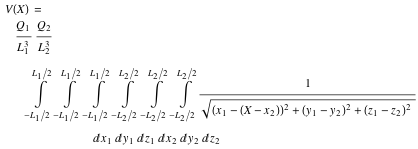(In the following calculations, we will skip the constant [with respect to X] prefactors Q1 L1-3 Q2 L2-3 or Q1 Q2 if not needed.)

Approaching this integral head-on doing one integral after another is possible, but a very tedious and time-consuming operation. Instead, to avoid having to carry out a nested six-dimensional integral, we remember the Laplace transform of 1 / √s.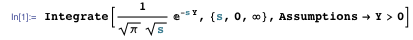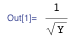Meaning that, modulo a numeric factor, the inverse of the square root function is self-reciprocal with respect to the Laplace transform.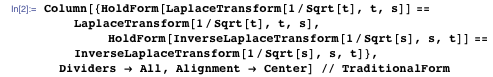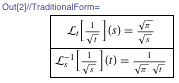Using this integral transform for the term ((x1 – (Xx2))2 + (y1y2)2 + (z1z2)2)½ changes the nested six-dimensional integral into three factors of double integrals. (Only one of the double integrals depends on X; this means the generalization to two four-dimensional cubes is straightforward at this point.) We calculate the function Vs that has to be integrated over s to obtain the full interaction energy. The three double integrals can all be carried out in closed form, and only a one-dimensional integral over s remains to be carried out.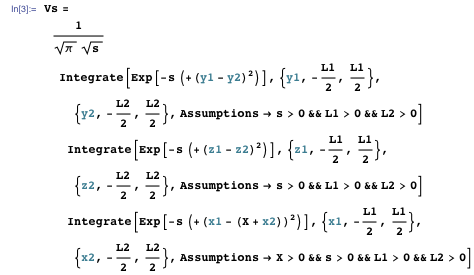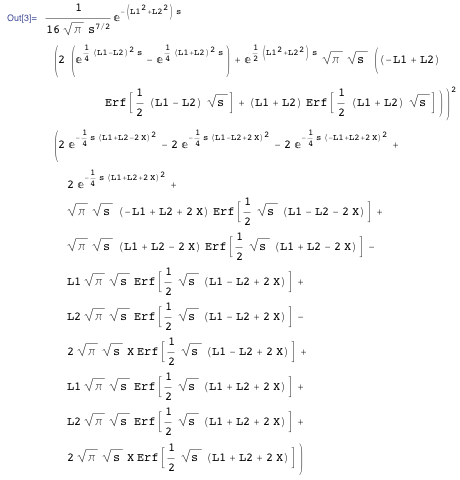Now we have to carry out the remaining integral over s. The forms of the last expressions first suggest a change of variable sq = s½ to get a nicer-looking integrand.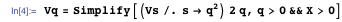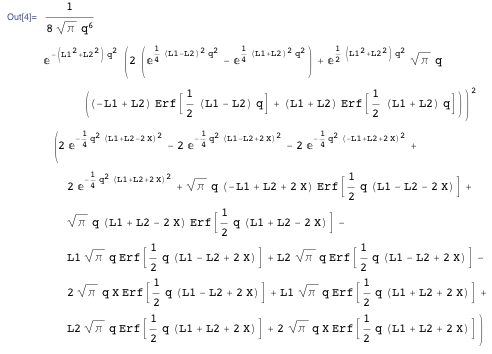The factor q-5 in the last expression suggests we carry out partial integrations, so we define a rule to carry out the partial integrations and apply this rule recursively to remove all higher negative powers of q. (We skip the fully integrated parts of the partial integration because they all vanish.)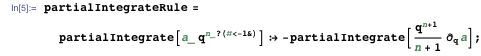And we define some functions to apply this rule to sums and products with numeric factors.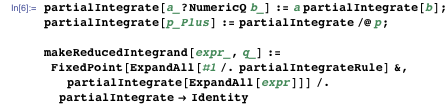The resulting expression now has more terms, but a simpler structure. A single term in Vq will result in many more terms after partial integration. This process removes all terms containing q-5, q-4, q-3, and q-2 and only keeps terms containing q-1. Here is an example of partially integrating out one summand out of the 264 summands.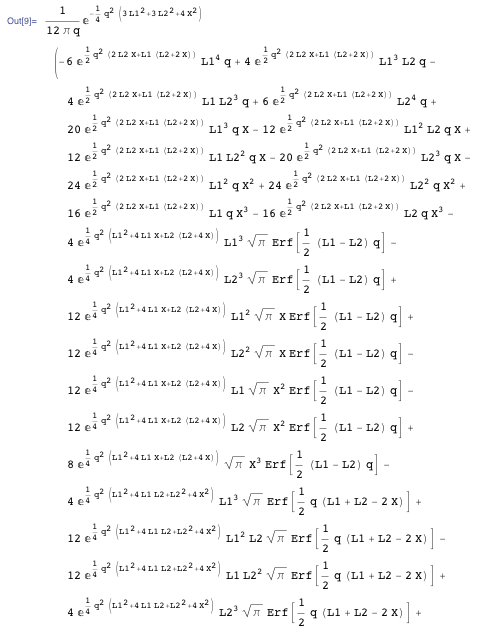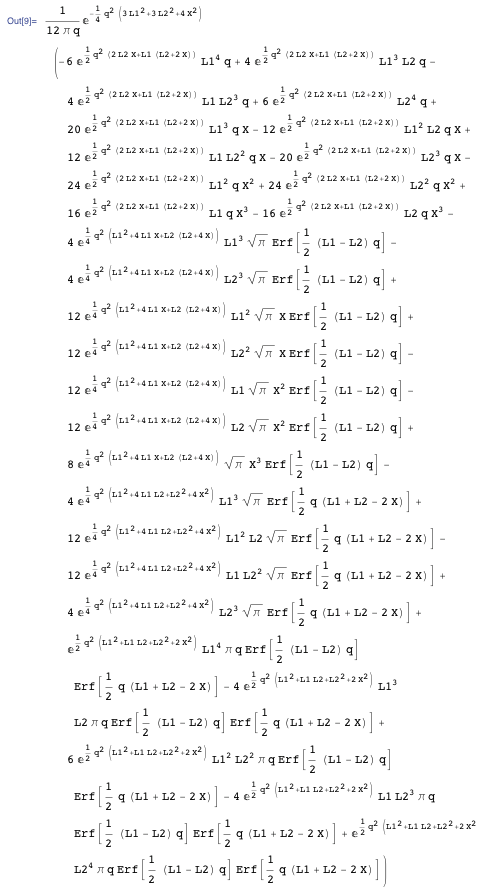Carrying out the partial integrations increases the size of our q-integrands from 264 to 1,196.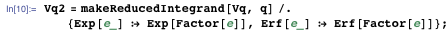Here are three of them randomly selected.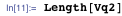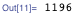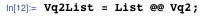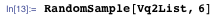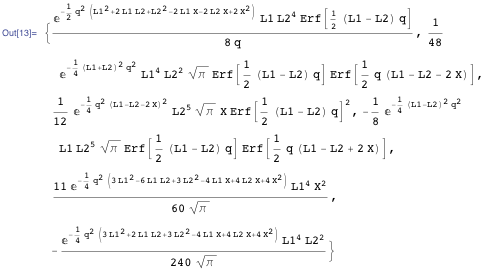A quick inspection of the terms shows that there are only four different types with respect to their q-dependence, with at most a q-1 factor. As our goal is to carry out the remaining integral over q, we define a quick pattern-matched integration function for the four types of integrands that occur in Vq2List (this will be much faster than using Integrate). One is just a Gaussian integral, and the other three integrals contain error functions multiplied by a Gaussian.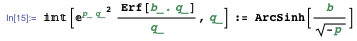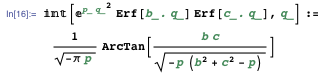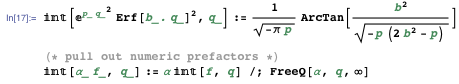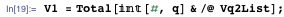Now we have a closed form for the potential. We carry out a quick numerical check of the integration by comparing the integration with a numerically carried out integral over q: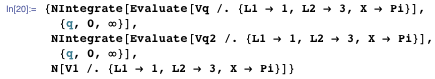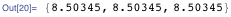Now we can easily calculate the resulting force. The result is relatively large. Inspecting the explicit form shows terms containing arcsinh and terms containing arctan. We simplify these terms individually: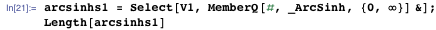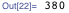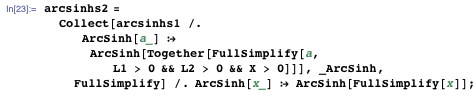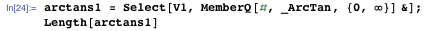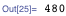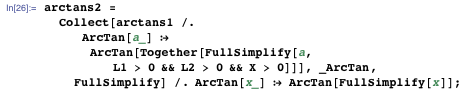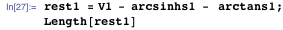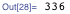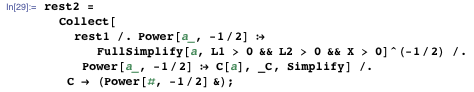The resulting expression is now smaller by more than a factor of 10.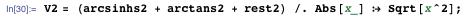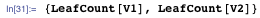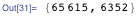It is still large, but manageable. Here are some of the 68 summands of V2 shown.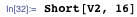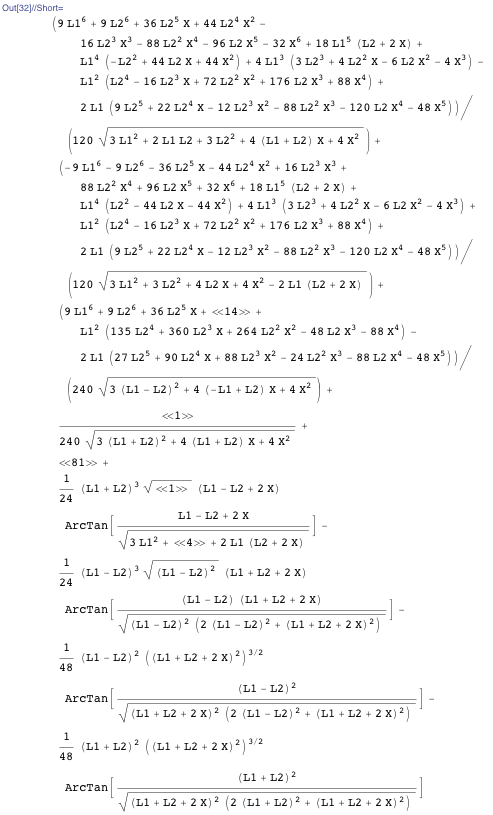Here are two plots of the total interaction energy and the force between the two cubes as a function of the edge length L2 of the second cube and the separation distance X. (We assume the first cube has unit edge length.)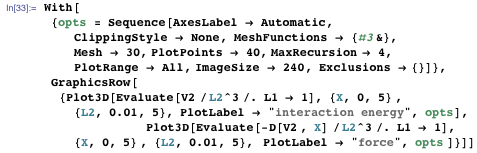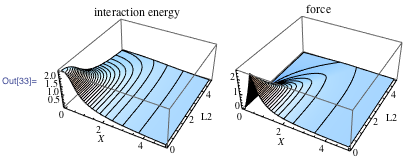The last graphic of the force shows the intuitive, to-be-expected behavior: For large separation distances X, the force decreases; for zero separation (concentric cubes), the force vanishes because of symmetry. And the sharp peak at X = 1/2 for small L2 is the maximum of the field strength at the center of a face of the unit cube.

Let’s have a quick look at the behavior of two cubes separated by a large distance. The term proportional X5 gives the first correction to the Coulomb law.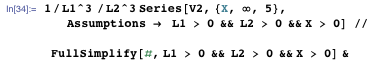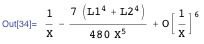As a quick check, we compare these leading terms with an integration over the series of the integrand.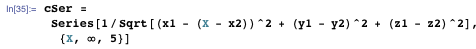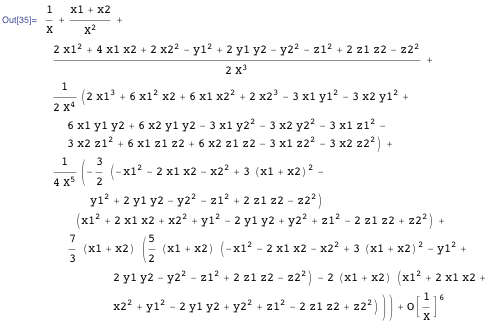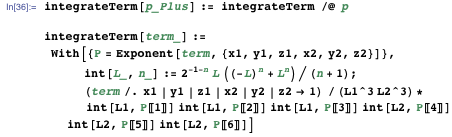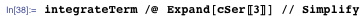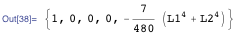Now, for the rest, let’s specialize to two cubes of equal edge length. Because of the presence of removable singularities, we cannot just substitute L2L1, but have to be slightly more careful. Making series expansions, it turns out that all indeterminate terms vanish in the limit L2L1.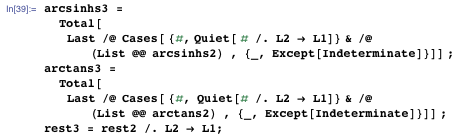This gives the following final result for the interaction potential of two identical cubes separated by a distance X. After some more simplifications, we get a result that fits on a single page. (We now include the prefactors [L-3]2.)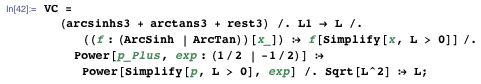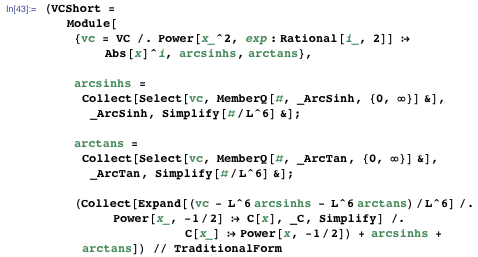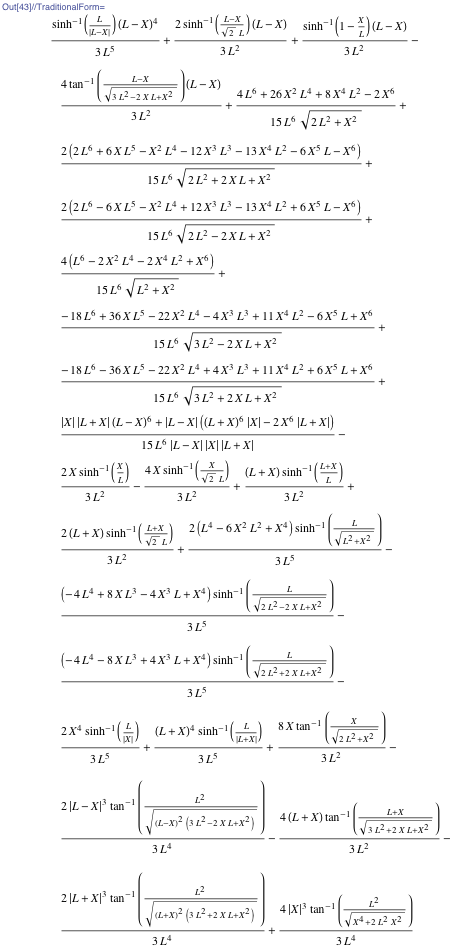The last expression has the form Vc = L-1 f(x) where the dimensionless x is defined as x = X/L. Here is a quick look at the function f(x) and its first two derivatives. The second derivative is no longer a smooth function at x = 1.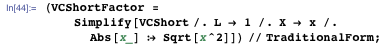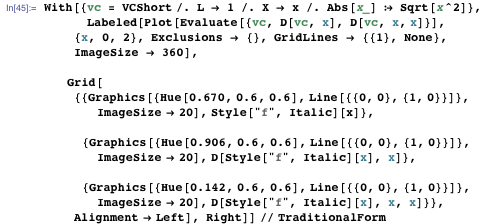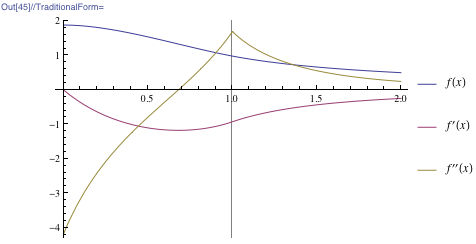And these are the asymptotic behaviors of f(x) at x = 0 and x = &#8734.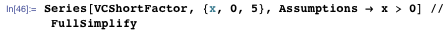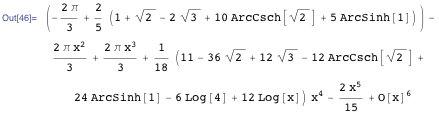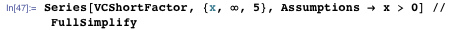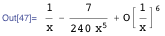In the series expansion around x = 1, we see the cusp of the second derivative reflected in the different values of the coefficient of (x – 1)3.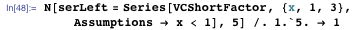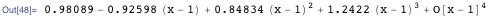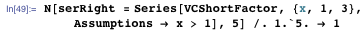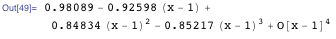The difference of the coefficients is just 2π/3.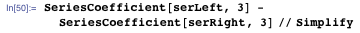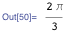We obtain the force between the two cubes by differentiating VC with respect to X. (Analogous to the well-known Newtonian force law between two homogeneous spherical objects, a natural name for this distance-dependent force law would be the Cubonian force law.)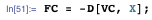And here is the exact expression for the force between two cubes touching each other along a face (meaning X = L). We use Series instead of substitution in FC due to indeterminate expressions arising in the substitution process.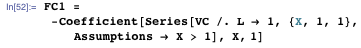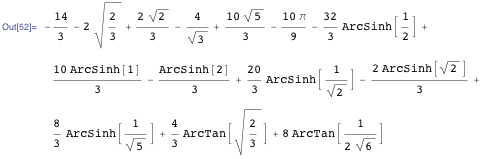And here is the numerical value we were looking for with 100 digits.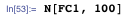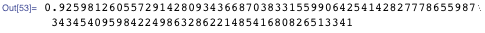There are various ways to represent this expression; here are some of them.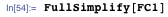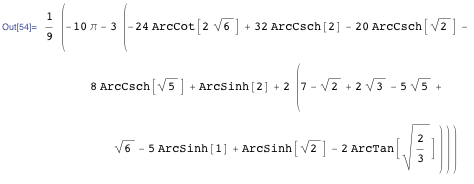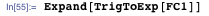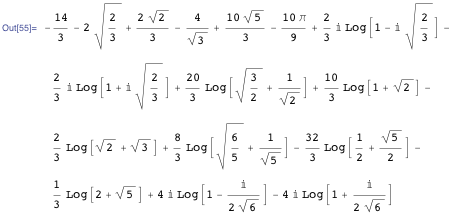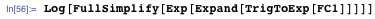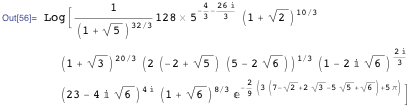Here are some more root reduced versions that better show the algebraic and transcendental part of the result.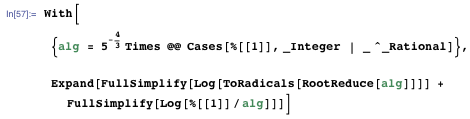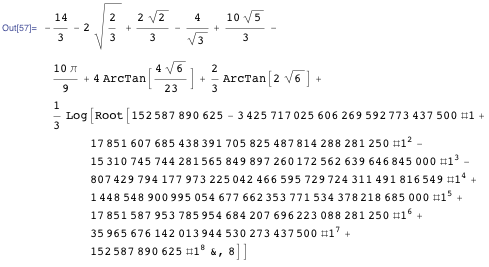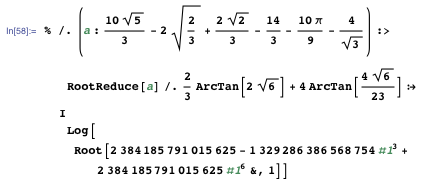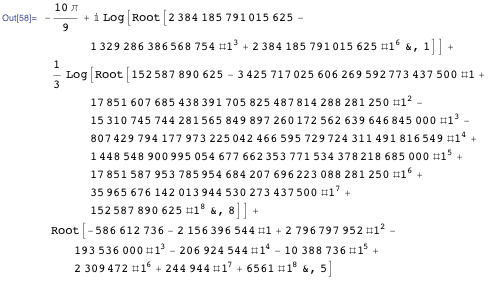Having the force between the two cubes as a function of the distance, a natural question to ask is: What is the force between two penetrable cubes, and how does it compare to the force between two spheres? The force between two penetrable, homogeneously charged spheres was calculated by Kermode, Mustafa, and Rowley. (We again ignore prefactors).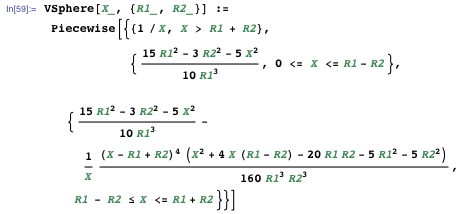We will use a sphere with the same volume as a unit cube.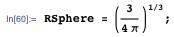Here are plots of the total interaction energy and the force between the two spheres. The blue curves are for the two cubes and the red curves for the two spheres. (The two vertical lines indicate the sphere radius and the half edge lengths.)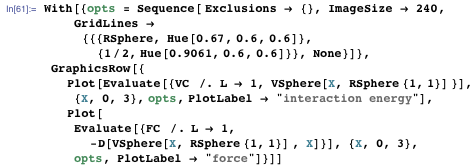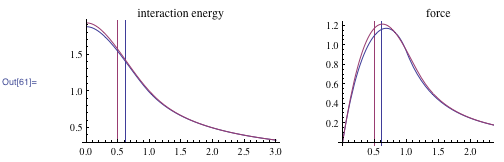We see that the maximum force between two cubes is slightly larger than the maximum force between two spheres of equal volume. The maximal force between two equal spheres occurs when the two sphere centers are separated by a distance equal to the sphere’s radius.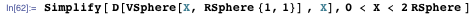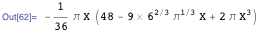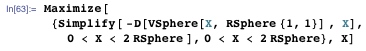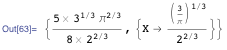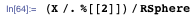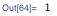The maximum force between two cubes occurs when the two cube centers are separated by about 68% of the cube edge length, and the maximal force between two cubes in this case is about 3.5% smaller than the maximal force between two spheres of equal volume.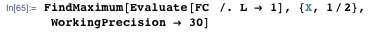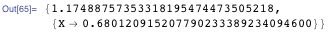So, now that we have the distance-dependent Cubonian force between two cubes, what can we calculate with it? For instance, we could build a soft-shell Newtonian cradle using charged, penetrable cubes.

We assume the strings to be very long so that the cubes all move in 1D and model the gravitational force as a linear restoring force to the cube’s resting positions.

We assume unit cubes positioned initially at positions xn] = n Δ for some given initial spacing Δ.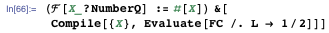Here is an example (download the CDF at the end of the post to interact with it):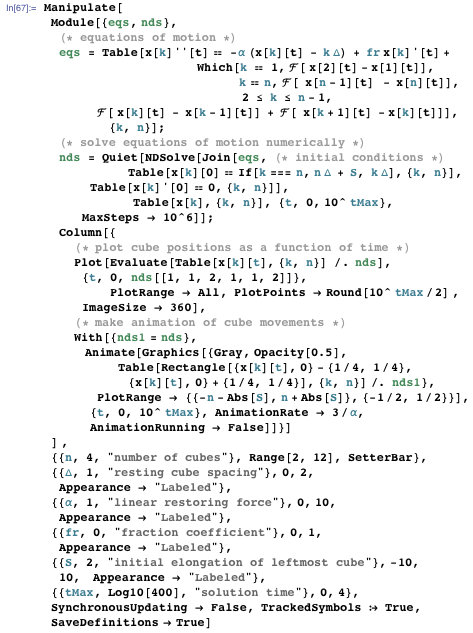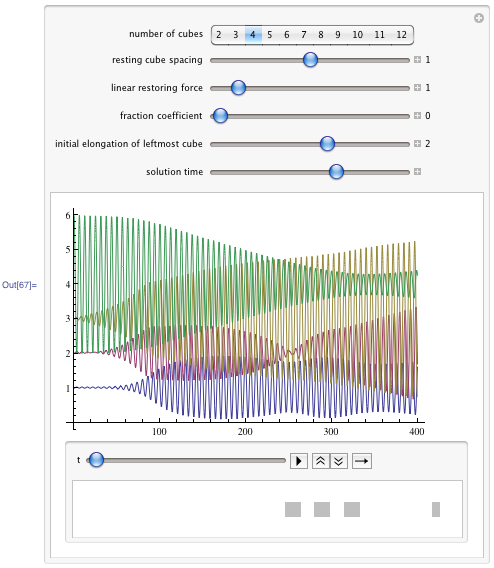Because the cubes are penetrable, the initially elongated cube carries out about 50 oscillations through the other cubes before the neighboring tubes start to move with a larger amplitude.